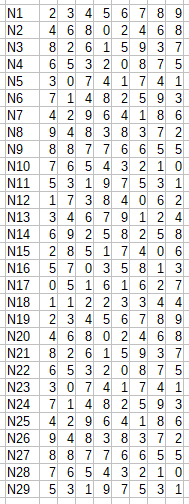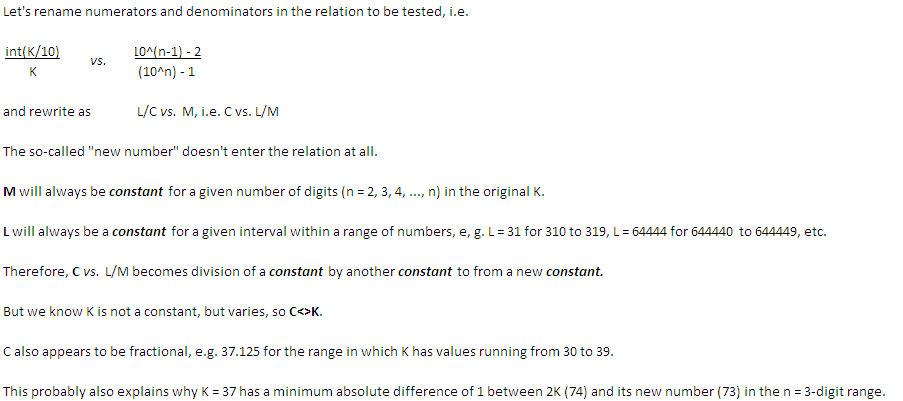BrainDen.com - Brain Teasers
• 1

# Find the missing value

## Question

I have in mind a number which, when you remove the units digit and place it at the front, gives the same result as multiplying the original number by 2. Am I telling the truth?

## Recommended Posts

• 1
Spoiler

Call the original number N and the new number M. Define N1 as the units digit of N, N2 as the tens digit of N, etc. If you use the same numbering system for M then the fact that M = 2N means that M1 = 2N1 mod 10, M2 = 2N2 mod 10 + int(N1/5), M3 = 2N3 mod 10 + int(N2/5), etc. And the fact that you can move the units digit of N to the front to also generate M means that M1 = N2, M2 = N3, … Mmax = N1.

Combining the facts that M1 = 2N1 mod 10 and M1 = N2 means that N2 = 2N1 mod 10

M2 = 2N2 mod 10 + int(N1/5) and M2 = N3 means N3 = 2N2 mod 10 + int(N1/5)

M3 = 2N3 mod 10 + int(N2/5)  …

Well, I could keep writing all that stuff out, but you get the idea. If you’re given the ones digit, you can solve for the rest of the digits. So we can just go through all the possible values for the ones digit and find out if we can generate a number that fits the description of the problem. Note that we can skip the cases where N1 = 0 or N1 = 1 because in those cases it would be impossible for M (where N1 is now the lead digit) to be 2N. I wrote a spreadsheet to do those calculations for the various potential starting values N1 and will paste the results.Now we’re looking for a number where the original N1 digit (which becomes the Mmax digit) would be 2Nmax + int(Nmax-1/5) which is what you know must be true if M = 2N. In English: if N1 is even we’re looking for a number with an Nmax of N1/2 and Nmax-1 less than 5, and if N1 is odd then we’re looking for an Nmax of N1/2 rounded down and Nmax-1 of 5 or greater. So taking the case of N1 = 2, we can look down the list of subsequent digits until we find a potential Nmax where Nmax = 1 and Nmax-1 < 5, which occurs at digit 18. That gives the number 105263157894736842. And manually checking, we see that in fact moving the ones digit to the front gives the same value as multiplying the original number by 2, which is 210526315789473684. A similar thing can be done for the other potential starting units digits to find more numbers that would be a solution to the problem.

##### Share on other sites

• 0
Spoiler

For 11 up to 9999 it's a lie! After that, maybe too.

Some figures get close, however, e.g. 25, 37, 449, 4499, one or two off the mark..

##### Share on other sites

• 0
On 4/24/2019 at 6:59 AM, rocdocmac said:
Hide contents

For 11 up to 9999 it's a lie! After that, maybe too.

Some figures get close, however, e.g. 25, 37, 449, 4499, one or two off the mark..

you have not proven nor disproven this

##### Share on other sites

• 0

I know, but somebody will perhaps!

Spoiler

Used trial and error up to 50 000. Don't know how to prove it mathematically (yet). I believe the statement will only be true at infinity.

Edited by rocdocmac

• 0

##### Share on other sites

• 0

The way I see it.

Spoiler##### Share on other sites

• 0

More ideas ...

Spoiler##### Share on other sites

• 0
On 4/23/2019 at 9:26 AM, BMAD said:

I have in mind a number which, when you remove the units digit and place it at the front, gives the same result as multiplying the original number by 2. Am I telling the truth?

You could be telling the truth because such a number does exist. Of course I can't say with certainty that you're telling the truth because I don't know whether you actually have such a number in mind right now. Back in a bit with a description of how to handle this problem.

## Join the conversation

You can post now and register later. If you have an account, sign in now to post with your account.×   Pasted as rich text.   Paste as plain text instead

Only 75 emoji are allowed.

×   Your previous content has been restored.   Clear editor

×   You cannot paste images directly. Upload or insert images from URL.